# Applications of Dimensional Analysis

Application of Dimensional Analysis.

(1) To find the unit of a physical quantity in a given system of units : In a dimensional formula, replacing M, L and T by the fundamental units of the required system we can get the unit of a physical quantity. However, sometimes to this unit we further assign a specific name, e.g., Work = Force ´ Displacement

So            Dimensions of Work = [MLT–2] * [L]  = [ML2T–2]

And  its units in C.G.S. system will be g cm2/s2 which is called erg while in M.K.S. system will be kg m2/s2 which is called joule.

(2) To convert a physical quantity from one system to the other :

For a physical quantity   nu = constant

If a physical quantity X  has dimensional formula [MaLbTc] and if (derived) units of that physical quantity in two systems are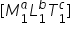and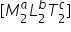n1 and n2 be the numerical values in the two systems respectively, then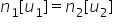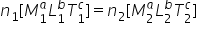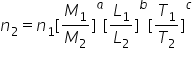where M1, L1 and T1­ are fundamental units of mass, length and time in the first (known) system and M2, L2 and T2 are fundamental units of mass, length and time in the second (unknown) system. Thus knowing the values of fundamental units in two systems and numerical value in one system, the numerical value in other system can be evaluated.

Example : Conversion of Newton into Dyne.

Newton is the S.I. unit of force .Its dimensional formula is  [MLT–2].

So 1 N = 1 kg-m/ sec2

By using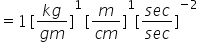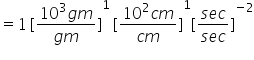=105

therefore 1 N = 105Dyne

(3) To check the dimensional correctness of a  physical relation : This is based on the principle of homogeneity. According to this principle the dimensions of each term on both sides of an equation must be  same.

Example :  S = ut- (1/2)at2

By substituting dimension of the physical quantities in the above relation –

[L] = [LT–1][T] – [LT–2][T2]

i.e.           [L] = [L] – [L]

As in the above equation dimensions of each term on both sides are same, so this equation is dimensionally correct. However, above equation is dimensionally correct and from equations of motion we know that

S = ut + (1/2)at2

(4) To derive new relations : If one knows the dependency of a physical quantity on other quantities and if the dependency is of the product type not of sum or subtraction or exponential or logarithmic, then using the method of dimensional analysis, relation between the quantities can be derived.

Example :  Time period of a simple pendulum.

Let time period of a simple pendulum is a function of mass of the bob (m), effective length (l), acceleration due to gravity (g) then assuming the function to be product of power function of m, l and g

i.e.,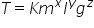; where K = dimensionless constant

If the above relation is dimensionally correct then by substituting the dimensions of quantities –

[T] = [M]x [L]y [LT–2]z

or  [M0L0T1]  = [MxLy+zT–2z]

Equating the exponents of similar quantities x = 0, y = 1/2 and z = – 1/2

So the required physical relation becomes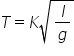The value of dimensionless constant is found (2π ) through experiments so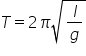Related Keywords